# New Rules:

## Quantum Circuits, Cellular Automata, Complexity and Chaos

austen.uk/slides/new-rules-tum

Austen Lamacraft and Pieter Claeys

### Rules of Life

• Each site either dead (0) or alive (1)

• Fate of cell determined by eight neighbors

1. Any live cell with two or three live neighbours survives
2. Any dead cell with three live neighbours becomes a live cell
3. All other live cells die in the next generation
• Complex behavior!

### Cellular Automata

• Dynamical systems with discrete space, time, and degrees of freedom

• Interesting for statistical physics:

• What kinds of dynamics may occur?
• How does dynamics determine thermodynamic behavior?

### Quantum Circuits

• A quantum analog of CAs

• Basis of “quantum supremacy” work by Google and others

### This talk

• What are the similarities and differences? Can we try the same tricks?

• When is quantum dynamics harder?

### Elementary cellular automata

• “Space” is one dimension with cells $x_n=0,1$ $n\in\mathbb{Z}$

• Update cells every time step depending on cells in neighborhood

• Neighborhood is cell and two neighbors for elementary CA
• Update specified by function

$$f:\{0,1\}^3\longrightarrow \{0,1\}.$$

$$x^{t+1}_{n} = f(x^{t}_{n-1},x^{t}_{n},x^{t}_{n+1})$$

• How many possible functions?

### Wolfram’s rules

• Domain of $f$ is $2^3=8$ possible values for three cells

• $2^8=256$ possible choices for the function $f$

• List outputs corresponding to inputs: 111, 110, … 000

111110101100011010001000
01101110
• Interpret as binary number: this one is Rule 110
• Many behaviors, from ordered (Rule 18) to chaotic (Rule 30)
• Rule 110 is capable of universal computation!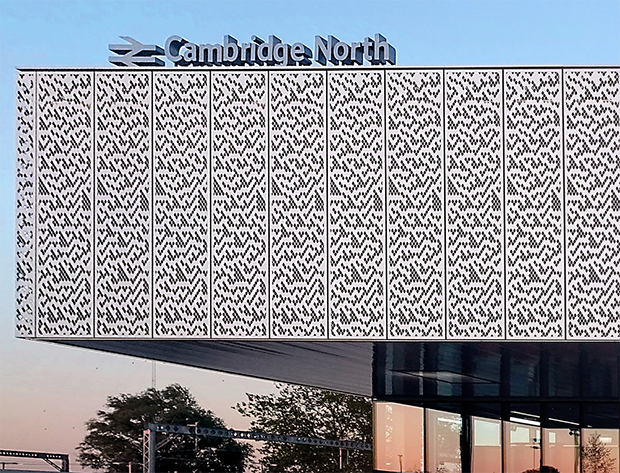### CAs as model physics

• Notion of a causal “light cone” (45 degree lines)

• Variety of possible behaviors: chaos, periodicity, …

### Chaos

• Rapid growth of small differences between two trajectories
• Smallest change: flip one site and monitor $z^t\equiv x^t\oplus y^t$

### Chaos phenomenology

• No exponential growth (c.f. Lyapunov exponent in continuous systems)

• Track number of differences (Hamming distance) between trajectories

• Propagating “front” cannot exceed “speed of light”: generally slower

### Theory?

• No chance of solving the dynamics of any one CA

• Looking for generic properties: natural to consider ensembles

• of initial conditions
• of rules

### Probabilistic CA

• Choose rules iid for each site and instant
• Cell values are now white noise, but $z_t=x_t\oplus y_t$ revealing

• Fluctuations of front are larger and average speed $<$ maximum

### Reversibility

• No elementary CAs are reversible (bijective)! Requires size 4 neighborhood

• Reversibility is undecidable above one spatial dimension

• $∃$ reversible constructions

### Second order cellular automata

• Fredkin construction

$$x^{t+1}_{n} = f(x^{t}_{n-1},x^{t}_{n},x^{t}_{n+1}) + x^{t-1}_{n},\, \mod 2$$

### Example: Integrable Rule 54

$$x^{t+1}_{n} = x^{t}_{n-1} x^{t-1}_{n} + x^{t}_{n+1} + x^{t}_{n-1}x^{t}_{n+1}\, \mod 2$$

• Interpret as “tiling rules”
• Random tilings consistent with constraint have entropy $\propto L$

### Block cellular automaton

• Partition cells into blocks (Margolus neighborhoods)
• Apply invertible mapping to block
• Alternate overlapping partitions

### Spacetime representation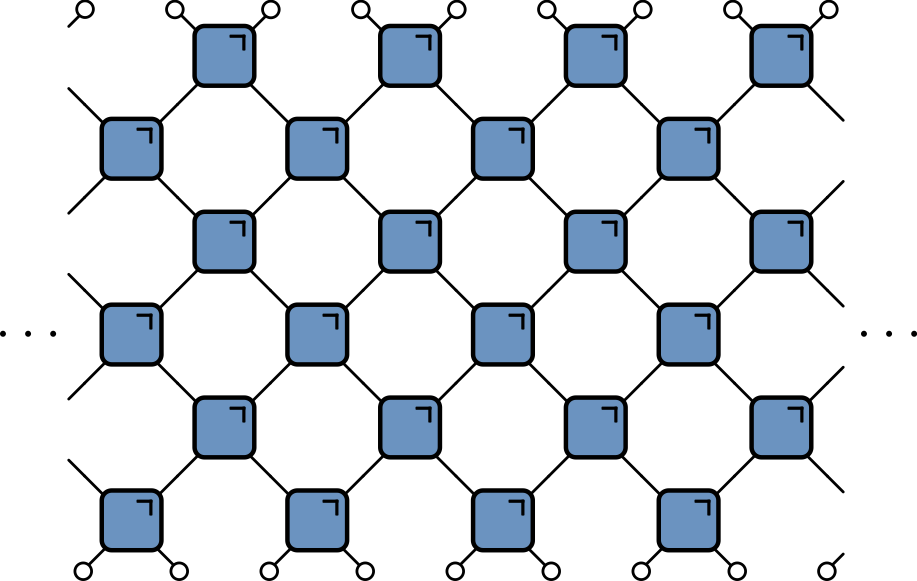• Blue squares: invertible mapping on states of two sites: 00, 01, 10, 11

## 24 reversible models

• Each block a permutation of 00, 01, 10, 11

• $4!=24$ blocks

• Order:

1. (1234)
2. (1243)
3. (1324), and so on
• Block 2 is the map $(00, 01, 10, 11) ⟶ (00, 10, 01, 11)$

• Exchange, or SWAP gate in quantum information

• Ensemble of block CAs similar to PCA

### Dual reversibility

• Can we find an ensemble where front propagates at maximal speed?

• Yes! Dual reversible blocks are bijections in both time and space

• There are 12 such blocks (out of 24)

• Ensemble is Markov in time and space: must have maximal velocity!

### Dynamics of difference?

• For $N=2$ dynamics of $z_t=x_t\oplus y_t$ independent of $x_t$ (or $y_t$)

• Recurrence time always $\propto L$ (instead of double exponential)

• Edge corresponds to the stationary state of a Markov process (c.f. Claeys and Lamacraft (2020) for quantum case)

### The linear block

$$(c,d) = f(a,b) = (a + b, a - b), \mod 3$$

• Original dual unitary circuit from Hosur et al.
• Unusual behavior of recurrence time
• For $L = 2\times 3^m$ have $T_\text{recur}=2L$
• Borsi and Pozsgay prove using Fourier analysis over finite fields

### Origin of “fractal” recurrence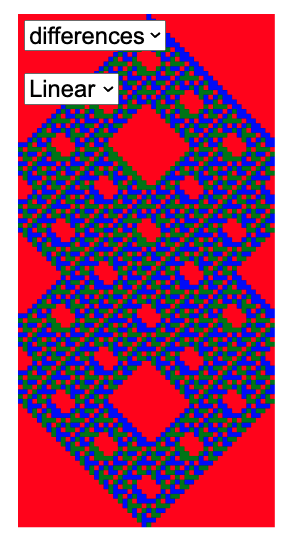$L=54=2\times 3^3$, $T_\text{recur}=2L=108$

### Mutual information

• Disjoint regions $A$ and $\bar A$: how much does one tell about the other?

• Use mutual information: measure of dependence of random variables

• Suggested in this context by Pizzi et al. (2022)

• MI defined as $$I(X;Y) \equiv S(X) + S(Y) - S(X,Y)$$

• $S(X)$ is entropy of $p_X(x)$; marginal distribution of $X$
• $S(Y)$ is entropy of $p_Y(y)$; marginal distribution of $Y$
• $S(X,Y)$ is entropy of joint distribution $p_{(X,Y)}(x,y)$
• Vanishes if $p_{(X,Y)}(x,y)=p_X(x)p_Y(y)$

### Simple example

• Suppose either $X=Y=1$ or $X=Y=0$, with equal probability

\begin{align} p_{(X,Y)}(0,0)&=p_{(X,Y)}(1,1)=1/2\\ p_{(X,Y)}(1,0)&=p_{(X,Y)}(0,1)=0 \end{align}

$$I(X;Y)=S(X) + S(Y) - S(X,Y)= 1+1-1=1 \text{ bit}$$

### Toy model

• Initial distribution factorizes over correlated pairs
• Apply SWAPs
• 1 bit MI for every pair with one member in $A$ and one in $\bar A$

$$I(A;\bar A) = \min(4\lfloor t/2\rfloor, |A|) \text{ bits}$$

• $|A|$ is (even) number of sites in $A$

• Total entropy conserved (c.f Liouville’s theorem)

• Entropy of initial distribution is half max, but entropy $S(A)$ saturates at maximal value (thermalization in time $\sim |A|/2$)

• This model is not so special! Any of the dual reversible blocks CAs behaves exactly the same!

### Graphical identities

For reversibility and dual reversibility

### Entropy from dual reversibility

• 8 sites share 4 bits of entropy

• After four steps increases to (maximum) 8 bits!

### Shallower…

• 8 sites share 4 bits of entropy

• After two steps increases to 6 bits!
• Entropies of $A$ and $\bar A$ subsystems behave just as in the toy model

$$I(A;\bar A) = \min(4\lfloor t/2\rfloor, |A|) \text{ bits}$$

• … for all dual reversible models!

### Classical dual reversible model

\begin{equation} \begin{aligned} \Phi_{\tau}\left(\mathbf{S}_{1}, \mathbf{S}_{2}\right) &=\frac{1}{\sigma^{2}+\tau^{2}}\left(\sigma^{2} \mathbf{S}_{1}+\tau^{2} \mathbf{S}_{2}+\tau \mathbf{S}_{1} \times \mathbf{S}_{2}, \sigma^{2} \mathbf{S}_{2}+\tau^{2} \mathbf{S}_{1}+\tau \mathbf{S}_{2} \times \mathbf{S}_{1}\right) \\ \sigma^{2} &:=\frac{1}{2}\left(1+\mathbf{S}_{1} \cdot \mathbf{S}_{2}\right) \end{aligned} \end{equation}

• MI has exactly the same behavior!

## Summary so far

• CAs as dynamical systems: chaotic fronts and information dynamics

• Dynamical ensembles as a theoretical tool

How can we extend these ideas to quantum systems?

### Bits to qubits

Block CAQuantum Circuit
Basic unitInvertible mapUnitary operator (gate)
Local variable$z_n \in \{0, 1\}$$\ket{\psi_n}\in \mathbb{C}^2 Global statez \in \{0,1\}^N$$\ket{\Psi(t)}\in \mathbb{C}^{2^N}$
SimulationEasyHard

### Why consider circuits?

• Model of universal quantum computation

• Example of discrete time, many body quantum dynamics

Everyone's doing it!

### Unitaries

• $n$-qubit unitary has matrix elements $U_{x_1\ldots x_n,x’_1,\ldots, x’_n}$ in computational basis $\ket{0}$, $\ket{1}$

• Unitarity means

$$\sum_{x_1’\ldots x_N’}U_{x_1\ldots x_n,x’_1,\ldots, x’_n} U^\dagger_{x’_1\ldots x’_n,x’’_1,\ldots, x’’_n}=\delta_{x_1,x_1’’}\ldots \delta_{x_N,x_N’’},$$

• But we’d like to avoid such awful looking expressions

### Everything’s a tensor!!

• General state of $N$ qubits is

$$\ket{\Psi} = \sum_{x_{1:N}\in \{0,1\}^N} \Psi_{x_1\ldots x_N}\ket{x_1}_1\ket{x_2}_2\cdots \ket{x_N}_N$$

• Write $\ket{x_1}_1\ket{x_2}_2\cdots \ket{x_N}_N =\ket{x_1\cdots x_N}=\ket{x_{1:N}}$ for brevity

• Operator on $N$ qubits has matrix elements

$$\mathcal{O}_{x_{1:N},x'_{1:N}} = \bra{x_{1:N}}\mathcal{O}\ket{x'_{1:N}}$$

### Brickwork unitary circuits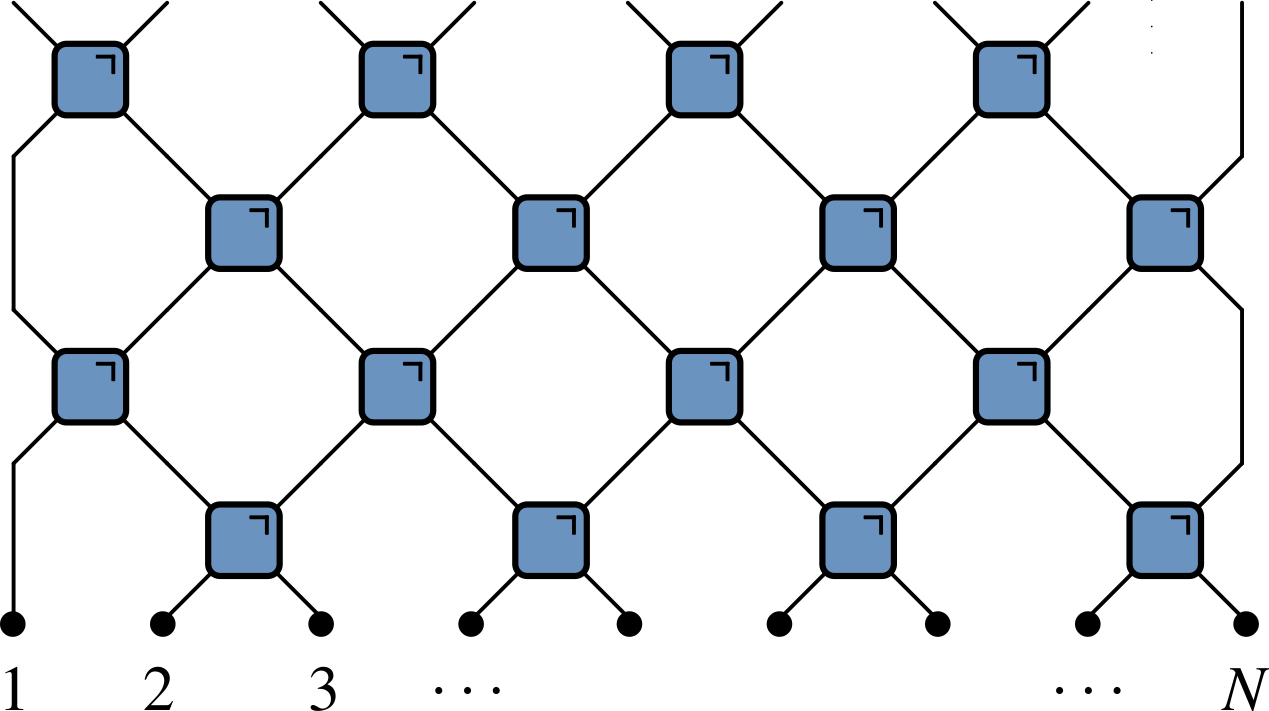• Have causality built in
• Quantum analog of (block) CAs

### Some gates

• Work in the basis $\ket{00}$, $\ket{01}$, $\ket{10}$, $\ket{11}$

• Simplest example: SWAP gate

$$\operatorname{SWAP}=\begin{pmatrix} 1 & 0 & 0 & 0 \\ 0 & 0 & 1 & 0 \\ 0 & 1 & 0 & 0 \\ 0 & 0 & 0 & 1 \end{pmatrix}$$

• Switches states. Takes product state to product state

$$\operatorname{SWAP}\ket{10} = \ket{01}$$

### Square root of SWAP

$$\sqrt{\operatorname{SWAP}}=\begin{pmatrix} 1 & 0 & 0 & 0 \\ 0 & \frac{1}{2}(1+i) & \frac{1}{2}(1-i) & 0 \\ 0 & \frac{1}{2}(1-i) & \frac{1}{2}(1+i) & 0 \\ 0 & 0 & 0 & 1 \end{pmatrix}.$$

• Generates entanglement (non product state)

$$\sqrt{\operatorname{SWAP}}\ket{10} = \frac{1}{2}\left[(1+i)\ket{10}+(1-i)\ket{01}\right]$$

• $\sqrt{\operatorname{SWAP}}$ and single qubit unitaries are universal gate set

### Gate notation

• We need both $U$s and $U^\dagger$s (e.g. for $\mathcal{O}(t)=U^\dagger(t)\mathcal{O}U(t)$)

• Much better!

### Hype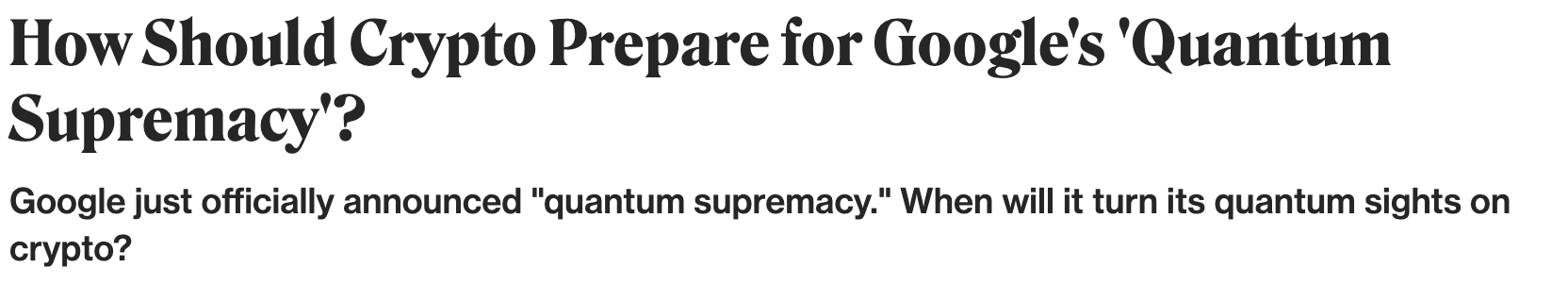### Computational complexity• Normally matrix-vector multiplication is $O(\operatorname{dim}^2)=2^{2N}$

• Gates are sparse so $O(\operatorname{dim})=2^{N}$, but still exponentially hard

• For low depth $T<N$ move horizontally instead

### Expectation values

• Evaluate $\bra{\Psi}\mathcal{O}\ket{\Psi}=\bra{\Psi_0}\mathcal{U}^\dagger\mathcal{O}\mathcal{U}\ket{\Psi_0}$ for local $\mathcal{O}$

### Folded picture

• After folding, lines correspond to two indices / 4 dimensions

### Unitarity in folded picture

• Circle denotes $\delta_{ab}$

### $\bra{\Psi}\mathcal{O}\ket{\Psi}$ in folded picture

• Emergence of “light cone”

### Reduced density matrix

• Expectation values in region $A$ evaluated using reduced density matrix

$$\rho_A = \operatorname{tr}_{\bar A}\left[\ket{\Psi}\bra{\Psi}\right]=\operatorname{tr}_{\bar A}\left[\mathcal{U}\ket{\Psi_0}\bra{\Psi_0}\mathcal{U}^\dagger\right]$$

### Entanglement entropy

• $\rho_A$ very useful for quantifying entanglement

• If $\ket{\Psi} = \ket{\psi}_A \otimes \ket{\phi}_{\bar A}$ then $\rho_A = \ket{\psi}_A\bra{\psi}_A$

• Any deviation from product state leads to mixed density matrix

• Quantify by entropy of $\rho_A$ (the entanglement entropy)

$$S_A \equiv -\operatorname{tr}\left[\rho_A\log \rho_A\right].$$

### Toy model revisted

• Each pair in Bell state $\ket{\Phi^+}_{2n, 2n+1} = \frac{1}{\sqrt{2}}\left[\ket{0}_{2n}\ket{0}_{2n+1}+ \ket{1}_{2n}\ket{1}_{2n+1}\right]$

• Reduced density matrix for one member: $\operatorname{tr}_{2}\left[\ket{\Phi^+}_{12}\bra{\Phi^+}_{12}\right] = \frac{1}{2}\mathbb{1}_1$

• Entanglement entropy of 1 bit

• For a Bell pair consisting of qubits at sites $m$ and $n$:

• If $n\in A$, $m\in\bar A$, $\rho_A$ has factor $\mathbb{1}_n$.

• If $m, n\in A$ they contribute a factor $\ket{\Phi^+}_{nm}\bra{\Phi^+}_{nm}$ (pure)

• Only first case contributes to $S_A = \min(4\lfloor t/2\rfloor, |A|) \text{ bits}$

• Just like mutual information in classical version!

### Dual unitary gates

• Exactly the same behavior for all unitaries satisfying
• c.f. dual reversible CAs

### The dual unitary family

• $4\times 4$ unitaries are 16-dimensional

• Family of dual unitaries is 14-dimensional

• Includes kicked Ising model at particular values of couplings

• Dual unitaries not “integrable” but have enough structure to allow many calculations

## $\rho_A$ via dual unitarity

• 8 sites; 4 layers

• $\rho_A$ is unitary transformation of

$$\mathbb{1}\otimes\mathbb{1}\otimes\mathbb{1}\otimes\mathbb{1}\otimes\mathbb{1}\otimes\mathbb{1}\otimes\mathbb{1}\otimes\mathbb{1}$$

## Shallower…

• $\rho_A$ is unitary transformation of

$$\mathbb{1}\otimes\mathbb{1}\ket{\Phi^+}\bra{\Phi^+}\otimes\ket{\Phi^+}\bra{\Phi^+}\otimes\mathbb{1}\otimes\mathbb{1}$$

### General case

• RDM is unitary transformation of

$$\rho_0=\overbrace{\frac{\mathbb{1}}{2}\otimes \frac{\mathbb{1}}{2} \cdots }^{t-1} \otimes\overbrace{\ket{\Phi^+}\bra{\Phi^+} \cdots }^{N_A/2-t+1 } \otimes \overbrace{\frac{\mathbb{1}}{2}\otimes \frac{\mathbb{1}}{2} \cdots }^{t-1}$$

• RDM has $2^{\min(2t-2,N_A)}$ non-zero eigenvalues all equal to $\left(\frac{1}{2}\right)^{\min(2t-2,N_A)}$

• Converse – maximal entanglement growth implies dual unitary gates – recently proved by Zhou and Harrow (2022)

## Thermalization

• After $N_A/2 + 1$ steps, reduced density matrix is $\propto \mathbb{1}$

• All expectations (with $A$) take on infinite temperature value

## Floquet theory: kicked Ising model

• Time dependent Hamiltonian with kicks at $t=0,1,2,\ldots$.

\begin{aligned} H_{\text{KIM}}(t) = H_\text{I}[\mathbf{h}] + \sum_{m}\delta(t-n)H_\text{K}\\ H_\text{I}[\mathbf{h}]=\sum_{j=1}^L\left[J Z_j Z_{j+1} + h_j Z_j\right],\qquad H_\text{K} &= b\sum_{j=1}^L X_j, \end{aligned}

• “Stroboscopic” form of $U(t)=\mathcal{T}\exp\left[-i\int^t H_{\text{KIM}}(t’) dt’\right]$

\begin{aligned} U(n_+) &= \left[U(1_+)\right]^n,\qquad U(1_-) = K I_\mathbf{h}\\ I_\mathbf{h} &= e^{-iH_\text{I}[\mathbf{h}]}, \qquad K = e^{-iH_\text{K}} \end{aligned}

## KIM as a circuit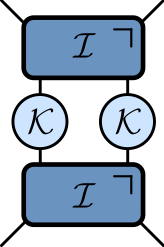\begin{aligned} \mathcal{K} &= \exp\left[-i b X\right]\\ \mathcal{I} &= \exp\left[-iJ Z_1 Z_2 -i \left(h_1 Z_1 + h_2 Z_2\right)/2\right]. \end{aligned}

### Entanglement Growth for Self-Dual KIM

$$\lim_{L\to\infty} S_A =\min(2t-2,N_A)\log 2,$$

• Any $h_j$; initial $Z_j$ product state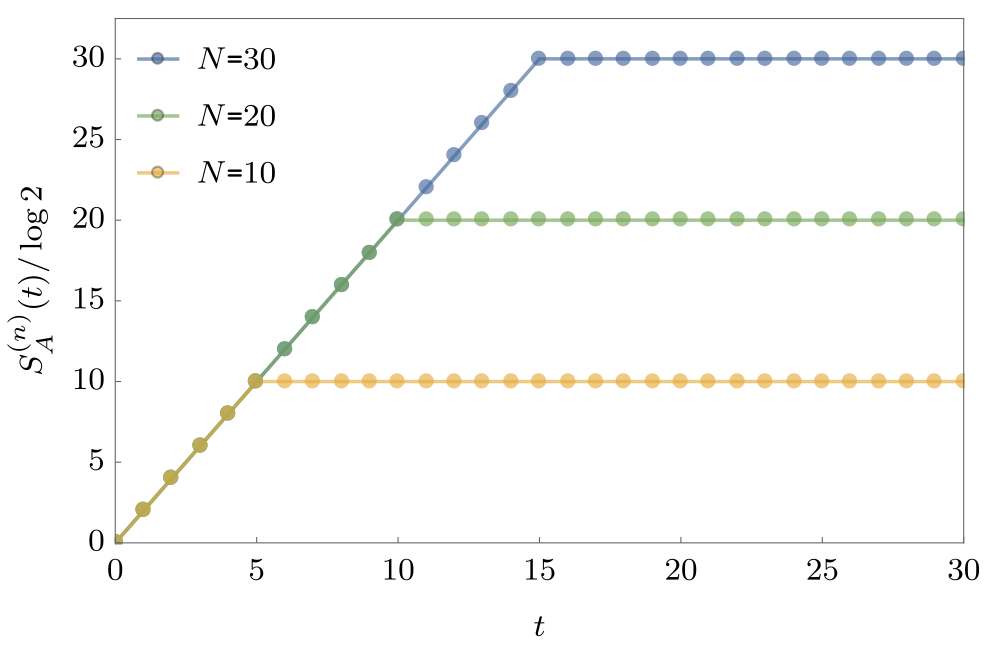## Dual unitarity

• Recall KIM has circuit representation\begin{aligned} \mathcal{K} &= \exp\left[-i b X\right]\\ \mathcal{I} &= \exp\left[-iJ Z_1 Z_2 -i \left(h_1 Z_1 + h_2 Z_2\right)/2\right]. \end{aligned}

• At $|J|=|b|=\pi/4$ model is dual unitary

## ‘KIM’ property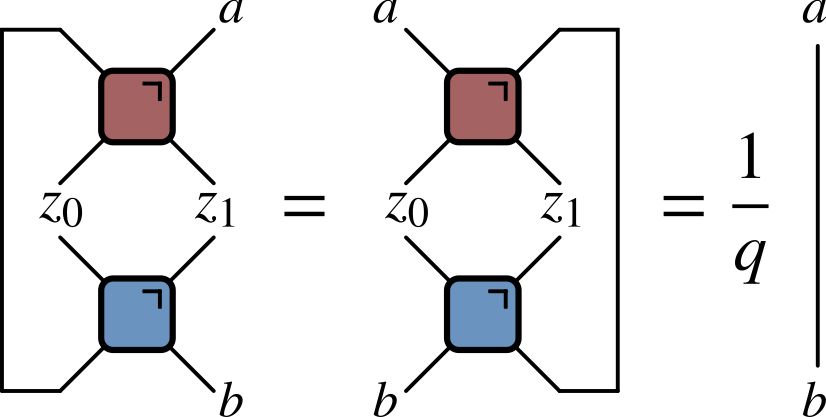• ($q=2$ here) Not satisfied by e.g. $\operatorname{SWAP}$

• Maps product states to maximally entangled (Bell) states

• Product initial states also work for KIM!

• Heisenberg picture: $Z_n(t)=\mathcal{U}^\dagger(t)Z_n \mathcal{U}(t)$

• Might use $Z_n(t)$ to evaluate correlation $\langle Z_n(t)Z_m(0) \rangle$

• How does $Z_n(t)$ look?

### Expansion in operator basis

• Expand $Z_n(t)$ in products of local operators $X_m$, $Y_m$, $Z_m$, $\mathbb{1}_m$

• Typical term $\sim \mathbb{1}_1\otimes \cdots X_{8}\otimes Y_{9} \otimes Z_{10}\cdots \otimes\mathbb{1}_N$

$$Z_n(t)= \sum_{\mu_{1:N}=\{0,1,2,3\}^N} \mathcal{C}_{\mu_{1:N}}(t) \sigma_1^{\mu_1}\otimes\cdots\otimes \sigma_N^{\mu_N},\qquad \sigma^\mu = (\mathbb{1},X,Y,Z)$$

As time progresses two things (tend to) increase:

1. The number of sites $\neq\mathbb{1}$ (known as operator spreading)
2. The number of different contributions (or operator entanglement)
• Operator spreading closely analogous to chaotic fronts in CAs

• Introduce ensemble of random circuits. $\mathcal{C}_{\mu_{1:N}}(t)$ become random

• Fluctuating signs mean $\langle Z_n(t)Z_m(0) \rangle$ will tend to average to zero

• c.f. a single PCA trajectory appears as white noise

### Out of time order correlator

$$\operatorname{OTOC}_{nm}(t) \equiv \langle Z_n(t)Z_m(0)Z_n(t)Z_m(0)\rangle.$$

• In terms of operator expansion

$$\operatorname{OTOC}_{nm}(t)\propto \sum_{\mu_{1:N}}\mathcal{C}_{\mu_{1:N}}^2(t)\left[\delta_{\mu_m,0}+\delta_{\mu_m,3}-\delta_{\mu_m,1}-\delta_{\mu_m,2}\right].$$`

• $\operatorname{OTOC}_{nm}(t)\neq 1$ when operator $Z_n(t)$ spreads from site $n$ to $m$

• Characteristic speed of propagation is “butterfly velocity” $v_\text{B}$

• OTOC quantum analog of bitstring differences $z_t=x_t\oplus y_t$ in CAs.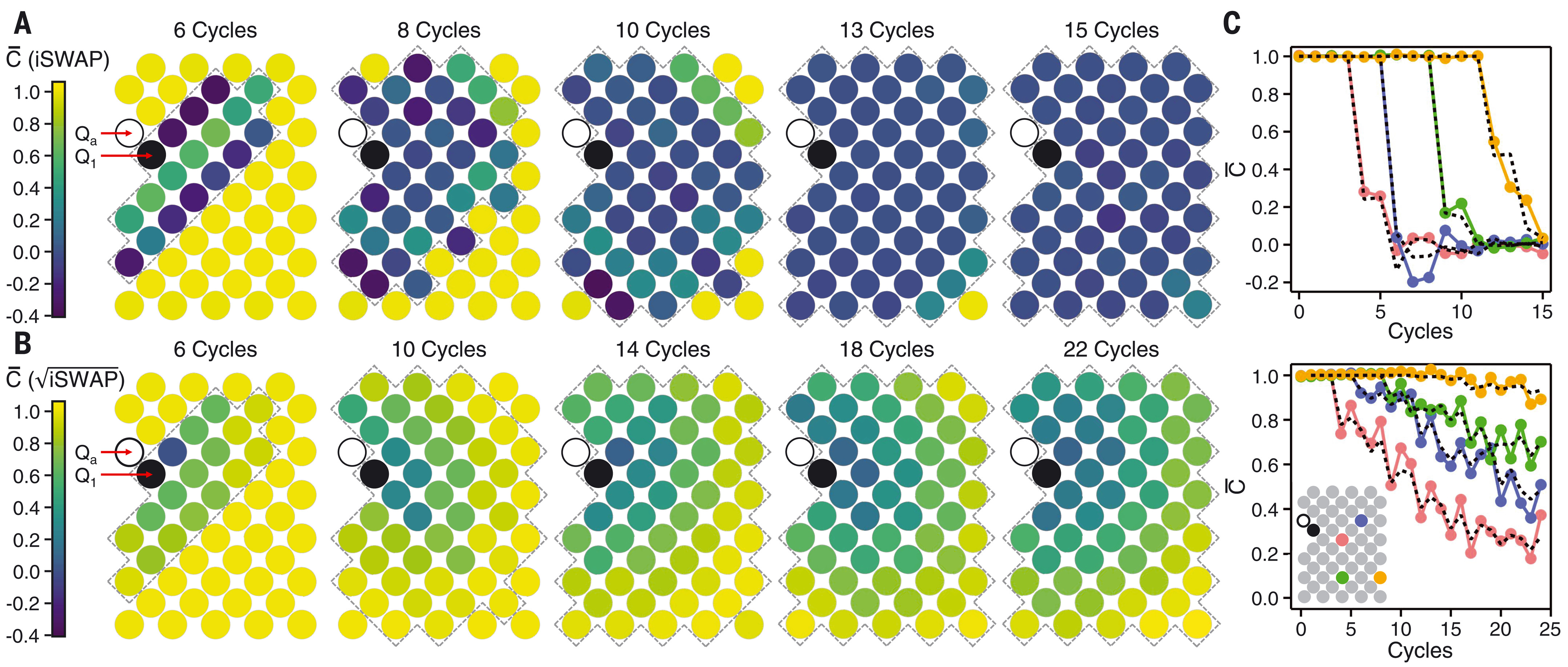The measured OTOC for $i\operatorname{SWAP}$ gates (top) and $\sqrt{i\operatorname{SWAP}}$ (bottom) after averaging over single qubit gates.

• $\overline{\operatorname{OTOC}}$ can be expressed as a Markov process

• Efficiently calculate using Monte Carlo simulations

Aren't quantum computers supposed to do things that classical computers find hard?

• Averaging is what enables efficient classical algorithms

• For a given circuit (no averaging), no probabilistic interpretation

### Frontier: measurements

• Unitary evolution not the only game in town!

• We can also measure, which we expect to reduce entanglement

• Consider measurements with certain rate and density in space

• All states purify, but on exponentially long times below transition

### Classical analogy?

• Interesting variation on PCA: choose output $1$ with probability $p$

• $p\neq 1/2$ makes dynamics less one-to-one. What happens?

### Phase transition

• For $0.25\lesssim p\lesssim 0.75$ front propagates to infinity

• Outside this region, front dies out

• In finite system two copies always merge after exponentially long time (“bad luck”)

### Markov chain on $z^t\equiv x^t\oplus y^t$

• $z^{t+1}_{n}=1$ only if at least one of $z^t_{n\pm 1}=1$

### Classical version of MIPT?

• Measurements purify state; analogous to non-injective rules in CA

• It was a surprise that a mixed state survives finite measurement rate

• But… a chaotic front survives non-injective rules (up to a point)

## Summary of analogies

Cellular AutomataQuantum Circuits
Chaos diagonisticDifference $z^t=x^t\oplus y^t$OTOC $\langle Z_n(t)Z_m(0)Z_n(t)Z_m(0)\rangle$